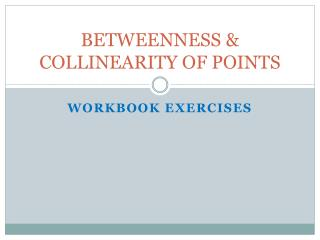DownloadDownload PresentationBETWEENNESS & COLLINEARITY OF POINTS

# BETWEENNESS & COLLINEARITY OF POINTS

Télécharger la présentation## BETWEENNESS & COLLINEARITY OF POINTS

- - - - - - - - - - - - - - - - - - - - - - - - - - - E N D - - - - - - - - - - - - - - - - - - - - - - - - - - -
##### Presentation Transcript

1. BETWEENNESS & COLLINEARITY OF POINTS WORKBOOK EXERCISES

2. A. Use the number line to find the measure of line segments. A B C D E F G H I J K -10 -8 -6 -4 -2 0 2 4 6 8 10 • 1. /A B/= 6. /BF/= • 2. /AK/= 7. /BE/ + /DG/= • 3. / JB/= 8. /AK/-/HK/= • 4. /HC/= 9. /JE/./DI/= • 5. /FK/= 10. /DH/÷/IK/=

3. B. Answer the following questions. • 1. A, B & C are the three collinear points on a line. Determine which point is between the other if their coordinates are -10,2 and-1 respectively. • 2. Points A,B & C are collinear points, B is between A and C. Find the distance of AB if AC = 40 and BC= 15. • 3. X,Y & Z are collinear points, /XZ/ +/YZ/ = /XY/ which point is between the other two points. • 4. Point B is between A and C, the coordinates of A & C are -6 and 8 respectively. What is the coordinate of B if AB = 6 & BC = 8.

4. B. Answer the following questions. • 5. A, B & C are the three collinear points. The coordinate of A is -6 and the coordinate of C is 20. What is the coordinate of B if AB = BC? • 6. Given the figure below. • A. If DF = 30, DE = x +5 & EF = 2x -2, find the value of x, the length of DE and EF. • B. If DF =65, DE = 3x +5 & EF = 2x +10, find the value of x, the length of DE and EF. D E F

5. BETWEENNESS & COLLINEARITY OF POINTS EXERCISES( PARALLEL QUESTIONS)

6. A. Use the number line to find the measure of line segments. A B C D E F G H I J K -10 -8 -6 -4 -2 0 2 4 6 8 10 • 1. /A B/= 6. /BF/= • 2. /AK/= 7. /BE/ + /DG/= • 3. / JB/= 8. /AK/-/HK/= • 4. /HC/= 9. /JE/./DI/= • 5. /FK/= 10. /DH/÷/IK/=

7. B. Answer the following questions. • 1. P, Q & R are the three collinear points on a line. Determine which point is between the other if their coordinates are -15, -20 and 5 respectively. • 2. Points A,B & S are collinear points, B is between A and S. Find the distance of BS if AS = 15 and AB= 5. • 3. G, M & A are collinear points, /GM/ +/MA/ = /GA/ which point is between the other two points. • 4. Point M is between U and I, the coordinates of U & I are 11 and -4 respectively. What is the coordinate of M if MU = 6.

8. B. Answer the following questions. • 5. P, Q & R are the three collinear points. The coordinate of P is -5 and the coordinate of R is 15. What is the coordinate of Q if PQ = RQ? • 6. Given the figure below. • A. If DF = 30, DE = x +5 & EF = 2x -2, find the value of x, the length of DE and EF. • B. If DF =65, DE = 3x +5 & EF = 2x +10, find the value of x, the length of DE and EF. D E F